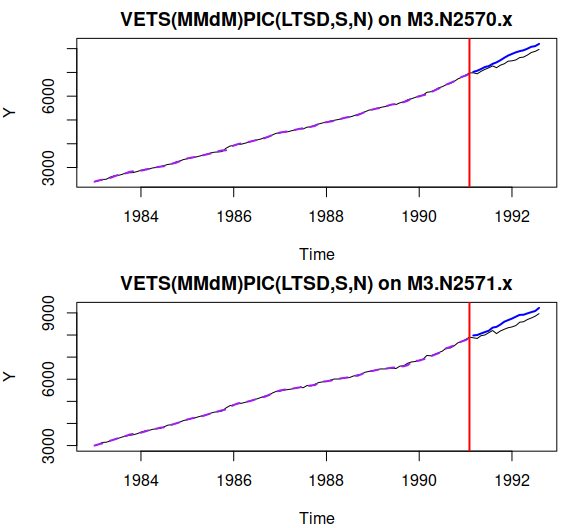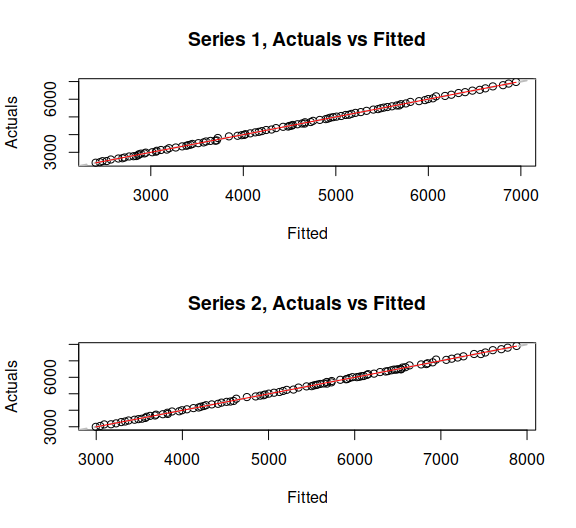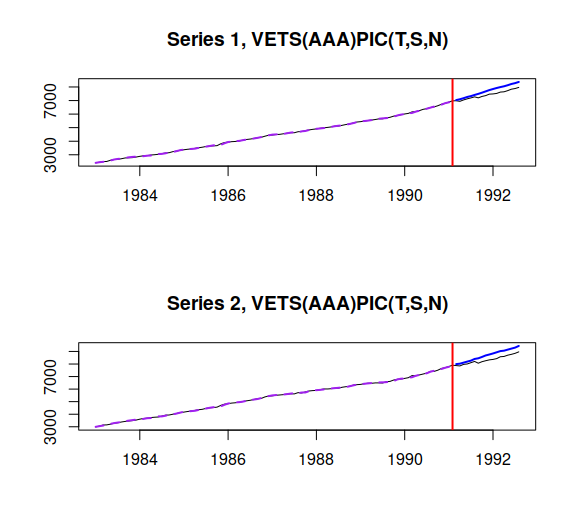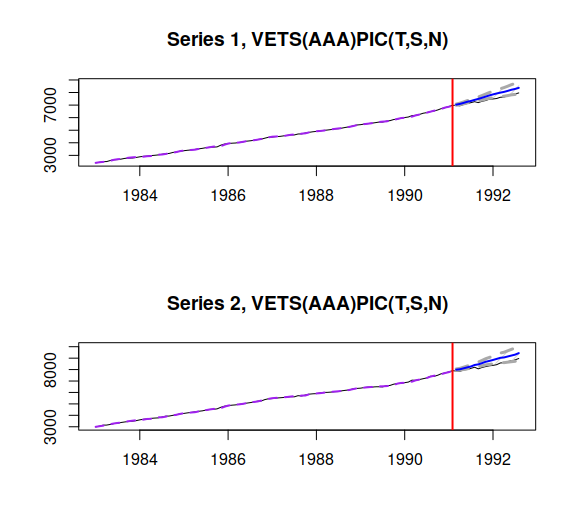# vets() - Vector ETS

#### 2021-05-15

This vignette covers vets() function, which is a part of legion package. In this vignette we will use data from Mcomp package, so it is advised to install it.

require(Mcomp)
require(legion)

We will use to time series from the M3 united in a vector:

Y <- cbind(M3$N2570$x,M3$N2571$x);

vets() function implements Vector ETS from Svetunkov, Chen, and Boylan (2021). This is a model that introduces two dimensional taxonomy: the original ETS one from Hyndman et al. (2008) and the extended for the restrictions on Parameters, Initials and Components (PIC) of the model. By default the function makes smoothing and dampening parameters common between different products and forces a common seasonal initials, resulting in ETS-PIC(LTSD,S,N):

vetsModel <- vets(Y, "MMdM", h=18, holdout=TRUE, silent=FALSE)The output tells us how much time the estimation took, what model was estimated, the loss function type used and its value, general information about sample size, number of parameters, number of variates and degrees of freedom and finally the information criteria for the model. Using the latter, it is possible to select the most appropriate ETS model and PIC restrictions. However, this process might take a lot of time, so there is also auto.vets() function that does a stepwise selection of components and restrictions:

vetsModel <- auto.vets(Y, "PPP", h=18, holdout=TRUE, silent=FALSE)
## Selecting the best unrestricted model...
## Initial model is VETS(AAA), AICc is: 1897.587
## Testing initials restrictions... 14 %29 %43 %57 %71 %86 %100 %
## Initials restrictions model is (s), AICc is: 1758.495
## Testing parameters restrictions... 7 %13 %20 %27 %33 %40 %47 %53 %60 %67 %73 %80 %87 %93 %100 %
## Parameters restrictions model is (t), AICc is: 1750.633
## Testing components restrictions... 20 %40 %60 %80 %100 %
## Components restrictions model is (none), AICc is: 1750.633

model="PPP" tells function to select the best between pure additive and pure multiplicative models. This parameter also accepts model="XXX", selecting between pure additive and model="YYY", selecting between pure multiplicative models. Note that if you want to impose restrictions on the initials and components, then pure multiplicative models typically make more sense, aligning with the idea of percentage change of value, rather than the change in the units of data (as in additive models).

Some methods to consider when working with vets():

oldpar <- par(mfcol=c(2,1))
plot(vetsModel,1)
plot(vetsModel,7)
par(oldpar)modelType(vetsModel)
##  "AAA"
modelType(vetsModel,pic=TRUE)
##  "T,S,N"
actuals(vetsModel)
fitted(vetsModel)
residuals(vetsModel)

The forecasts can be produced using forecast() method:

vetsForecast <- forecast(vetsModel, h=18, interval="prediction")
oldpar <- par(mfcol=c(2,1))
plot(vetsForecast)
par(oldpar)The detailed explanation of the underlying model and possible restrictions are provided in Svetunkov, Chen, and Boylan (2021).